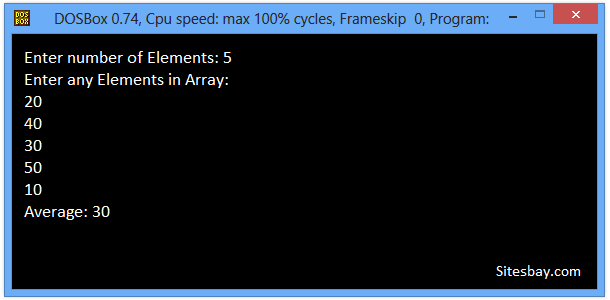# Find Average of Numbers Using Arrays Program in C++

## C++ Program to Find Average of Numbers Using Arrays

Average of numbers is calculated by adding all the numbers and then dividing the sum by count of numbers available.
In below code we receive integer input from user and storing it in array. Then we will find the sum of all array elements using for loop and finally calculate the average of N input numbers stored in an array.

To understand this code you need basic knowledge about Array in C++ and For Loop in C++.

## Example

```The numbers whose average is to be calculated are:
10, 20, 30, 40, 50
Sum of numbers = 150
Average of numbers = 150/5 = 30
```

## C++ Program to Find Average of Numbers Using Arrays

```#include<iostream.h>
#include<conio.h>

void main()
{
int i, count, sum, inputArray;
float average;
clrscr();

cout<<"Enter number of Elements: ";
cin >> count;

cout<<"Enter " << count << " any Elements in Array:\n";
for(i = 0; i < count; i++) {
cin >> inputArray[i];
}

sum = 0;
// Find sum of all array elements
for(i = 0; i < count; i++) {
sum += inputArray[i];
}

average = (float)sum / count;
cout << "Average: " << average;

getch();
}
```

## Output

```Enter number of Elements: 5
Enter any Elements in Array:
20
40
30
50
10
Average: 30
```## Pure VPN Privide Lowest Price VPN Just @ \$1.65. Per Month with Non Detected IP Lowest Price Non Detected IP VPN